## Example Questions

← Previous 1 3 4 5 6 7 8 9

### Example Question #2271 : Gmat Quantitative Reasoning

Find the length of the diagonal of square G.

I) The area of G is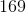fathoms squared.

II) The side length of G isfathoms.

Each statement alone is enough to solve the question.

Statement 2 is sufficient to solve the question, but statement 1 is not sufficient to solve the question.

Both statements taken together are sufficient to solve the problem.

Neither statement is sufficient to solve the question. More information is needed.

Statement 1 is sufficient to solve the question, but statement 2 is not sufficient to solve the question.

Each statement alone is enough to solve the question.

Explanation:

We can use the side length and the Pythagorean Theorem to find the diagonal of a square.

We can find side length from area, so we could solve this with either I or II.

### Example Question #2272 : Gmat Quantitative Reasoning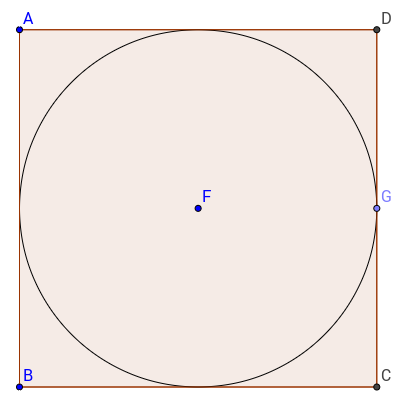The circle with centeris inscribed in square. What is the length of diagonal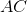?

(1) The area of the circle is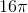.

(2) The side of the square is.

Statements 1 and 2 together are not sufficient.

Statement 2 alone is sufficient.

Both statements together are sufficient.

Statement 1 alone is sufficient.

Each statement alone is sufficient.

Each statement alone is sufficient.

Explanation:

The diagonal of the square can be calculated as long as we have any information about the lengths or area of the circle or of the square.

Statement 1, by giving us the area of the circle, allows us to find the radius of the circle, which is half the length of the side. Therefore statement 1 alone is sufficient.

Statement 2, by telling us the length of a side of the square is also sufficient, and would allow us to calculate the length of the diagonal.

Therefore, each statement alone is sufficient.

### Example Question #2273 : Gmat Quantitative Reasoning

On your college campus there is a square grassy area where people like to hangout and enjoy the sun. While walking with some friends, you decide to take the shortest distance to the corner of the square opposite from where you are. Find the distance you traveled.

I) The perimeter of the square ismeters.

II) The square covers an area of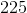square meters.

Either statement is sufficient to answer the question.

Statement II is sufficient to answer the question, but statement I is not sufficient to answer the question.

Statement I is sufficient to answer the question, but statement II is not sufficient to answer the question.

Both statements are needed to answer the question.

Either statement is sufficient to answer the question.

Explanation:

We are asked to find the length of a diagonal of a square.

We can do this if we have the side length. We can find side length from either perimeter or area.

From Statement I)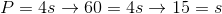In this case, our side length is 15 meters.

We can use this and Pythagorean Theorem or 45/45/90 triangles to find our diagonal.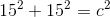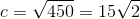From Statement II)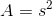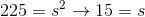From here, we can plug the side length into the Pythagorean Theorem like before and solve for the diagonal.

Therefore, either statement alone is sufficient to answer the question.

### Example Question #2274 : Gmat Quantitative Reasoning

Find the length of the diagonal of square A if the diagonal of square B is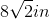.

1. The perimeter of square B is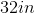2. The area of square A is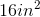Statement 1 alone is sufficient, but statement 2 alone is not sufficient to answer the question.

Statement 2 alone is sufficient, but statement 1 alone is not sufficient to answer the question.

Both statements taken together are sufficient to answer the question, but neither statement alone is sufficient.

Each statement alone is sufficient to answer the question.

Statements 1 and 2 are not sufficient, and additional data is needed to answer the question.

Statement 2 alone is sufficient, but statement 1 alone is not sufficient to answer the question.

Explanation:

Statement 1: The information provided would only be useful if the ratio of square A to square B was known.

Statement 2: We need the length of the square's side to find the length of the diagonal and we can use the area to solve for the length of the side.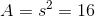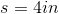Now we can find the diagonal: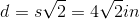### Example Question #2275 : Gmat Quantitative Reasoning

What is the length of the diagonal of the square?

1. The area of the square is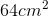.
2. The perimeter is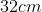.

Statement 2 alone is sufficient, but statement 1 alone is not sufficient to answer the question.

Statement 1 alone is sufficient, but statement 2 alone is not sufficient to answer the question.

Both statements taken together are sufficient to answer the question, but neither statement alone is sufficient.

Each statement alone is sufficient to answer the question.

Statements 1 and 2 are not sufficient, and additional data is needed to answer the question.

Each statement alone is sufficient to answer the question.

Explanation:

The length of the diagonal of a square is given by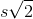, whererepresents the square's side. As such, we need the length of the square's side.

Statement 1: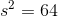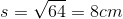Statement 2: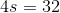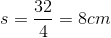Both statements provide us with the length of the square's side.

### Example Question #2276 : Gmat Quantitative Reasoning

The diagonal bracing of a square pallet measures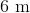. What is the area of the pallet?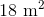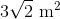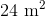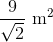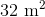Explanation: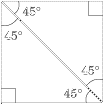To solve this problem, we must recognize that the diagonal bisector creates identical 45˚ - 45˚ - 90˚ right triangles. This means that, if the sides of the square arethen the diagonal must be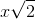. We can then set up the following equation: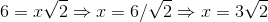If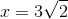then the area must be: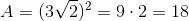### Example Question #1 : Dsq: Calculating The Length Of The Side Of A Square

Is Rectangle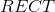a square?

Statement 1: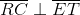Statement 2: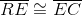Statement 2 ALONE is sufficient to answer the question, but Statement 1 ALONE is NOT sufficient to answer the question.

BOTH statements TOGETHER are insufficient to answer the question.

BOTH statements TOGETHER are sufficient to answer the question, but NEITHER statement ALONE is sufficient to answer the question.

EITHER statement ALONE is sufficient to answer the question.

Statement 1 ALONE is sufficient to answer the question, but Statement 2 ALONE is NOT sufficient to answer the question.

EITHER statement ALONE is sufficient to answer the question.

Explanation:

A rectangle, by definition, is a parallelogram. Statement 1 asserts that the diagonals of this parallelogram are perpendicular. Statement 2 asserts that adjacent sides of the parallelogram are congruent, so, since opposite sides are also congruent, this makes all four sides congruent. From either statement alone, it can be deduced that Rectangleis a rhombus. A figure that is a rectangle and a rhombus is by definition a square.

### Example Question #2 : Dsq: Calculating The Length Of The Side Of A Square

Find the side length of square R.

I) The area of square R is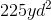.

II) The perimeter of square R is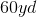.

Statement 2 is sufficient to solve the question, but statement 1 is not sufficient to solve the question.

Both statements taken together are sufficient to solve the question.

Neither statement is sufficient to solve the question. More information is needed.

Statement 1 is sufficient to solve the question, but statement 2 is not sufficient to solve the question.

Each statement alone is enough to solve the question.

Each statement alone is enough to solve the question.

Explanation:

Consider the following equations: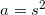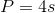Where a is area, p is perimeter, and s is side length

We can find the side length with either our area or our perimeter.

Thus, we only need one statment or the other.

### Example Question #3 : Dsq: Calculating The Length Of The Side Of A Square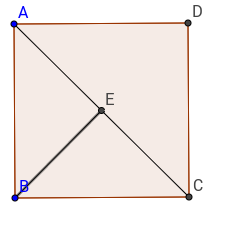What is the length of the side of square, knowing that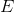is the midpoint of diagonal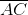?

(1)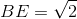(2)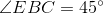Both statements together are sufficient

Statement 2 alone is sufficient

Statements 1 and 2 together are not sufficient

Statement 1 alone is sufficient

Each statement alone is sufficient

Statement 1 alone is sufficient

Explanation:

Since ABCD is a square, we just need to know the length of the diagonale to find the length of the side. BE is half the diagonal, therefore knowing its length would help us find the length of the sides.

Statement 1 tells us the length of BE, therefore, with the formula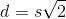whereis the diagonal andthe length of side, we can find the length of the side.

Statement 2 tells us that triangle AEB is isoceles, but it is something we could already have known from the beginning since we are told that E is the midpoint of the diagonal.

Therefore, statement 1 alone is sufficient.

### Example Question #4 : Dsq: Calculating The Length Of The Side Of A Square

Find the area of square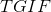.

I)has a diagonal of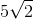inches.

II)has a perimeter ofinches.

Either statement alone is sufficient to answer the question.

Statement I is sufficient to answer the question, but Statement II is not sufficient to answer the question.

Statement II is sufficient to answer the question, but Statement I is not sufficient to answer the question.

Both statements together are needed to answer the question.

Either statement alone is sufficient to answer the question.

Explanation:

To find the area of a square we need to find its side length.

In a square, the diagonal allows us to find the other two sides. The diagonal of a square creates two 45/45/90 triangles with special side length ratios.

I) Gives us the diagonal, which we can use to find the side length, which will then help us find the area.

II) Perimeter of a square allows us to find side length, which in turn lets us find area.

So, either statement is sufficient.

← Previous 1 3 4 5 6 7 8 9

Tired of practice problems?

Try live online GMAT prep today.